### Home > CALC > Chapter 12 > Lesson 12.1.2 > Problem12-22

12-22.
1. Consider the infinite series below. For each, decide if it converges or diverges and justify your conclusion. State which test you used. Homework Help ✎

1.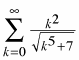2.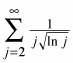3.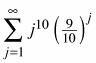4.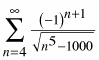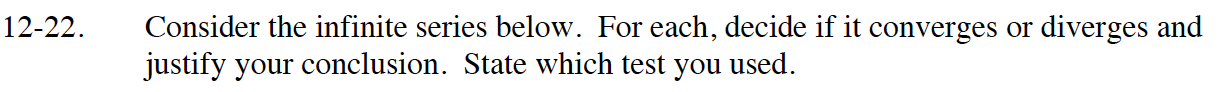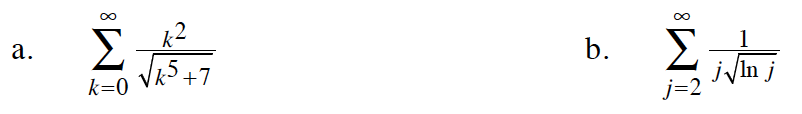Compare the argument of this summation to:

$\frac{k^2}{\sqrt{k^5}}=k^{-1/2}$

Use the Integral Test. To evaluate the integral, let u = ln(j).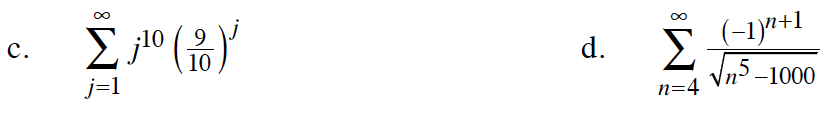Use the Ratio Test.

$\lim_{j\to\infty}\Bigg|\frac{j^{10}\Big(\frac{9}{10}\Big)^{j+1}}{j^{10}\Big(\frac{9}{10}\Big)^j}\Bigg|$

Compare this series to:

$\sum_{n=5}^{\infty}\frac{1}{n^2}$